NeurIPS2019 DISN

## 参考论文

DISN: Deep Implicit Surface Network for High-quality Single-view 3D Reconstruction

## Introduction## Method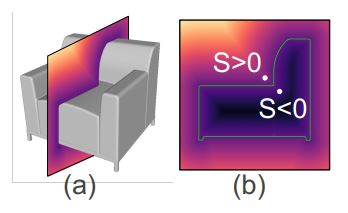SDF相当于一个连续的函数，将给定的空间中的点$p = (x, y, z) \in \mathbb{R}^3$映射到一个实数$s \in \mathbb{R}: s = SDF(p)$。s的绝对值表示点到曲面的距离，s的符号表示点在曲面外还是曲面内。则曲面$\mathcal{S}_0 = \{p | SDF(p) = 0\}$即可用于代表一个三维的物体。

### DISN: Deep Implicit Surface Network

DISN的整体结构如下图所示：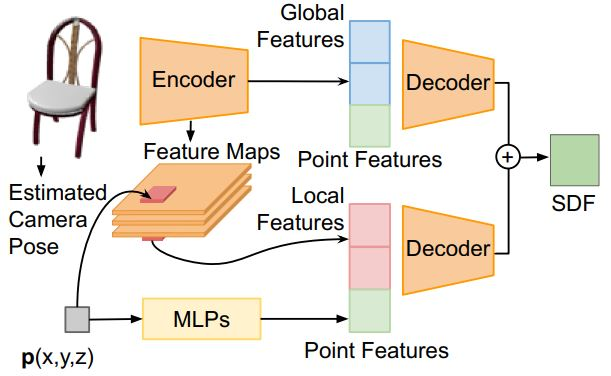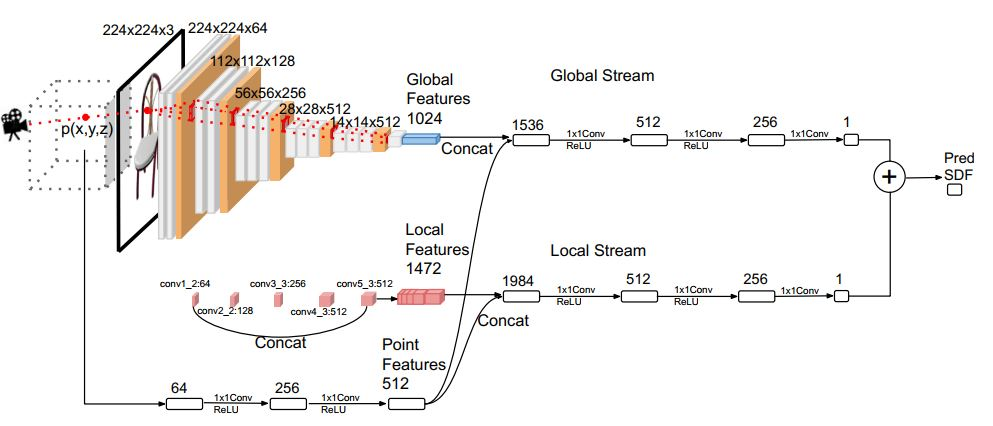DISN网络被分为两个部分：相机姿态估计以及SDF预测。首先，通过二维图像预测三维模型投影到二维图像所对应的相机参数，再通过预测的参数将每一个三维点投影到二维平面上并在每层上提取对应的局部特征。最后，DISN将根据多层的局部特征以及全局特征将给定的空间中的点解码为对应的SDF值。

#### Camera Pose Estimation

$\bm{R} = (\bm{R_x}, \bm{R_y}, \bm{R_z})^T \in \mathbb{R}^{3 \times 3}$

$\bm{R_x} = N(\bm{b_x}), \bm{R_z} = N(\bm{R_x} \times \bm{b_y}), \bm{R_y} = \bm{R_z} \times \bm{R_x}$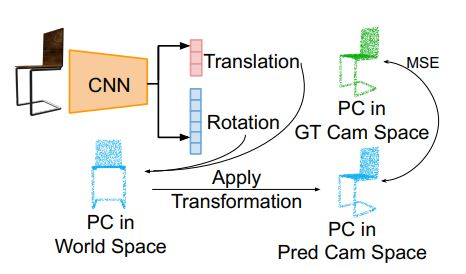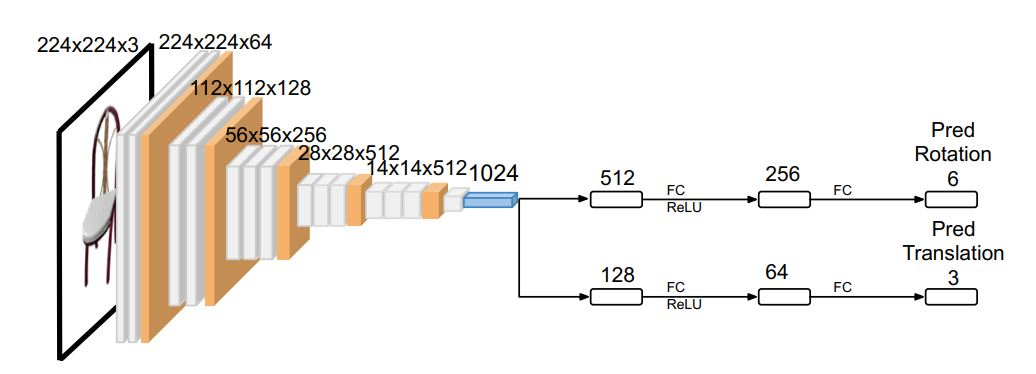$L_{cam} = \frac{\sum_{\bm{p}_w \in PC_w}||\bm{p}_G - (\bm{Rp}_w + \bm{t})||^2_2}{\sum_{\bm{p}_w \in PC_w}1}$

#### SDF Prediction with Deep Neural Network

Local Feature Extraction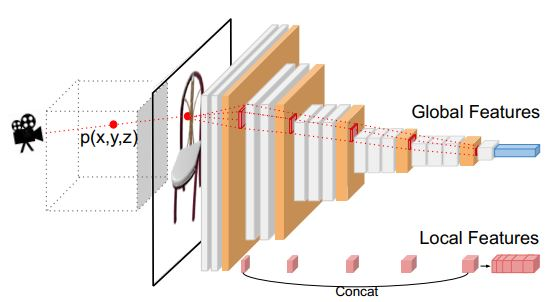Loss Function

$L_{SDF} = \sum_{p}m|f(I, \bm{p}) - SDF^I(p)|,$

$m = \left\{ \begin{array}{ll} m_1, & if \ SDF^I(\bm{p}) < \delta \\ m_2, & otherwise \end{array} \right.$